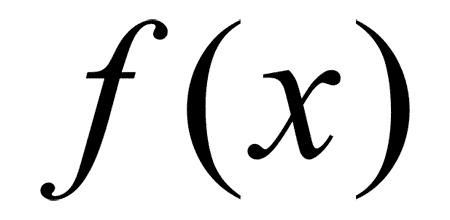# 函数式编程初探(1 + 2) * 3 – 4

var a = 1 + 2;

var b = a * 3;

var c = b – 4;

var result = subtract (multiply (add (1,2)， 3)， 4);

1. 函数是"第一等公民"

var print = function (i){ console.log (i);};

[1,2,3].forEach (print);

2. 只用"表达式"，不用"语句"

"表达式"（expression）是一个单纯的运算过程，总是有返回值；"语句"（statement）是执行某种操作，没有返回值。函数式编程要求，只使用表达式，不使用语句。也就是说，每一步都是单纯的运算，而且都有返回值。

3. 没有"副作用"

4. 不修改状态

function reverse (string) {

if (string.length == 0) {

return string;

} else {

return reverse (string.substring (1, string.length)) + string.substring (0, 1);

}

}

5. 引用透明

1. 代码简洁，开发快速

Paul Graham 在《黑客与画家》一书中写道：同样功能的程序，极端情况下，Lisp 代码的长度可能是C代码的二十分之一。

2. 接近自然语言，易于理解

subtract (multiply (add (1,2)， 3)， 4)

add (1,2) .multiply (3) .subtract (4)

merge ([1,2],[3,4]) .sort () .search ("2")

3. 更方便的代码管理

4. 易于"并发编程"

var s1 = Op1();

var s2 = Op2();

var s3 = concat (s1, s2);

5. 代码的热升级